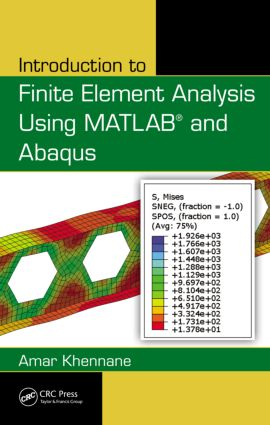# Introduction to Finite Element Analysis Using MATLAB® and Abaqus

## 1st Edition

CRC Press

487 pages | 360 B/W Illus.

##### Purchasing Options:\$ = USD
Hardback: 9781466580206
pub: 2013-06-10
\$170.00
x
eBook (VitalSource) : 9780429166433
pub: 2013-06-10
from \$28.98

FREE Standard Shipping!

### Description

There are some books that target the theory of the finite element, while others focus on the programming side of things. Introduction to Finite Element Analysis Using MATLAB® and Abaqus accomplishes both. This book teaches the first principles of the finite element method. It presents the theory of the finite element method while maintaining a balance between its mathematical formulation, programming implementation, and application using commercial software. The computer implementation is carried out using MATLAB, while the practical applications are carried out in both MATLAB and Abaqus. MATLAB is a high-level language specially designed for dealing with matrices, making it particularly suited for programming the finite element method, while Abaqus is a suite of commercial finite element software.

Includes more than 100 tables, photographs, and figures

Provides MATLAB codes to generate contour plots for sample results

Introduction to Finite Element Analysis Using MATLAB and Abaqus introduces and explains theory in each chapter, and provides corresponding examples. It offers introductory notes and provides matrix structural analysis for trusses, beams, and frames. The book examines the theories of stress and strain and the relationships between them. The author then covers weighted residual methods and finite element approximation and numerical integration. He presents the finite element formulation for plane stress/strain problems, introduces axisymmetric problems, and highlights the theory of plates. The text supplies step-by-step procedures for solving problems with Abaqus interactive and keyword editions. The described procedures are implemented as MATLAB codes and Abaqus files can be found on the CRC Press website.

### Reviews

"A very good introduction to the Finite Element Method with a balanced treatment of theory and implementation."

—F. Albermani, Reader in Structural Engineering, The University of Queensland, Australia

### Table of Contents

Introduction

Prologue

Finite Element Analysis and the User

Aim of the Book

Book Organization

Bar Element

Introduction

One-Dimensional Truss Element

Global Stiffness Matrix Assembly

Boundary Conditions

Solution of the System of Equations

Support Reactions

Members’ Forces

Computer Code: truss.m

Problems

Analysis of a Simple Truss with Abaqus

Beam Element

Introduction

Stiffness Matrix

Uniformly Distributed Loading

Internal Hinge

Computer Code: beam.m

Problems

Analysis of a Simple Beam with Abaqus

Rigid Jointed Frames

Introduction

Stiffness Matrix of a Beam–Column Element

Stiffness Matrix of a Beam–Column Element in the Presence of Hinged End

Global and Local Coordinate Systems

Global Stiffness Matrix Assembly and Solution for Unknown Displacements

Computer Code: frame.m

Analysis of a Simple Frame with Abaqus

Stress and Strain Analysis

Introduction

Stress Tensor

Deformation and Strain

Stress-Strain Constitutive Relations

Solved Problems

Weighted Residual Methods

Introduction

General Formulation

Galerkin Method

Weak Form

Integrating by Part over Two and Three Dimensions (Green Theorem)

Rayleigh Ritz Method

Finite Element Approximation

Introduction

General and Nodal Approximations

Finite Element Approximation

Basic Principles for the Construction of Trial Functions

Two-Dimensional Finite Element Approximation

Shape Functions of Some Classical Elements for C0 Problems

Numerical Integration

Introduction

Gauss Quadrature

Integration over a Reference Element

Integration over a Triangular Element

Solved Problems

Plane Problems

Introduction

Finite Element Formulation for Plane Problems

Spatial Discretization

Constant Strain Triangle

Linear Strain Triangle

The Bilinear Quadrilateral

The 8-Node Quadrilateral

Solved Problem with MATLAB

Axisymmetric Problems

Definition

Strain–Displacement Relationship

Stress–Strain Relations

Finite Element Formulation

Programming

Analysis with Abaqus Using the 8-Node Quadrilateral

Thin and Thick Plates

Introduction

Thin Plates

Thick Plate Theory or Mindlin Plate Theory

Linear Elastic Finite Element Analysis of Plates

Boundary Conditions

Computer Program for Thick Plates Using the 8-Node Quadrilateral

Analysis with Abaqus

Appendix A: List of MATLAB Modules and Functions

Appendix B: Statically Equivalent Nodal Forces

Appendix C: Index Notation and Transformation Laws for Tensors

References and Bibliography

Index

### About the Author

Dr. Amar Khennane is a senior lecturer in the School of Engineering and Information Technology at the University of New South Wales, Canberra, Australian Capital Territory, Australia. He earned his PhD in civil engineering from the University of Queensland, Australia; a Master of Science in structural engineering from Heriot-Watt University, United Kingdom; and a bachelor’s degree in civil engineering from the University of Tizi-Ouzou, Algeria. His teaching experience spans 20 years, and two continents. He has taught structural analysis, structural mechanics, and the finite element method at various universities.

### Subject Categories

##### BISAC Subject Codes/Headings:
TEC005000
TECHNOLOGY & ENGINEERING / Construction / General
TEC063000
TECHNOLOGY & ENGINEERING / Structural